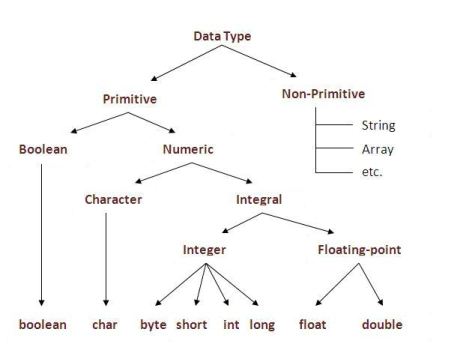## Basic Data Types and Variables in Java

Data Types: There are two types of data types:

###Primitive Data Types

These are the predefined data types. In Java, there are eight primitive data types, which are as follows:

 Data Types Default Values Range Default Size Example boolean False True/False 1 bit boolean b = True char ‘\u0000’ ‘\u0000’ to ‘\uffff’ 2 bytes char c=’A’ byte 0 -128  (-2^7) to 127 (2^7 -1) 1 byte byte  b = 12 short 0 -32,768 (-2^15)  to  32,767(2^15 -1) 2 bytes short s=1000 int 0 – 2,147,483,648 (-2^31)  to 2,147,483,647(2^31 -1) 2 bytes int i= 100000000 long 0L -9,223,372,036,854,775,808(-2^63)  to 9,223,372,036,854,775,807(2^63 -1) 8 bytes long l= 1000000L float 0.0f Single-precision 32-bit IEEE 754 floating point 4 bytes float f = 25.9f double 0.0d Double-precision 64-bit IEEE 754 floating point 8 bytes double d = 152.3

#### Variable

A variable is a memory address that can change, and if the memory address cannot change, then it is known as a constant. A variable is the name of the memory location where data is stored. Once a variable is stored with data, then space is allocated in memory. We can define a variable using a combination of numbers, letters, and the underscore character.

There are three types of variables: Local, instance, and static.• Local variable: The variable that we declare inside a method is called a local variable.

Example:

```public class intellipaatclass
{
public void method()
{
int a = 10;  // Local Variable
}
}
```
• Instance variable: The variable that we declare inside a class but outside the method is called an instance variable. It is not declared as static.

Example:

```public class intellipaatclass
{
int a = 10;  // Instance Variable
public void method()
{
}
}
```
• Static variable: The variable that we declare as static is called a static variable. It cannot be local.

Example:

```public class intellipaatclass
{
static int a = 10;  // Static Variable
public void method()
{
}
}
```

### Non-primitive Data Types

Non-primitive data types have instances like objects. They are also called reference types. We have a total of four types of non-primitive data types as given below:

• Classes
• Strings
• Interfaces
• Arrays

Let’s discuss these non-primitive data types one by one next.

#### 1. Classes

Classes are user-defined data types. They are the blueprint of objects. They have member variables and class methods.

Let’s have a look at a basic Java program to demonstrate classes:

```class Intellipaat {
int a,b,c;
void teach() {
System.out.println(“Intellipaat courses!”);
}
void learn() {
System.out.println(“Intellipaat courses!”);.
}
}
>/pre>```

#### 2. Strings

We know that generally, strings are a collection of characters. But in Java, a string is a completely different class. It is located in java.lang.String. Let’s have a look at these examples below: Example 1:

```String s=”Intellipaat courses!”;
String sub=s.substring(0,8);
System.out.println(sub);
```

Example 2:

```class TestStringConcatenation21{
public static void main(String args[]){
String a="Intellipaat"+" Courses";
System.out.println(a);//Intellipaat Courses
}  }
```

#### 3. Array

Arrays are used to store the same type of data in special memory locations. They are indexed. Indexing of arrays always starts from 0. In Java, we can dynamically allocate arrays.
Example:

```int arr[] = new int;
```

Here, storage space for 90 integers will be created.

Below is the Java program for demonstration of arrays:

```import java.io. * ;
import java.util. * ;
class Intellipaat {
public static void main(String[] args) throws IOException {
int i;
Scanner sc = new Scanner(System. in );
int arr[] = new int[] {
1,
2,
3,
4,
5,
6
};
int arr1[] = new int;
for (i = 0; i < 6; i++) {
arr1[i] = sc.nextInt();
}
System.out.println(“Previously assigned array”);
for (i = 0; i < 6; i++) {
System.out.println(arr[i]);
}
System.out.println("");
System.out.println("User entered array is:");
for (i = 0; i < 6; i++) {
System.out.println(arr1[i]);
}
}
}
```

#### 4. Java Interfaces

Interfaces are very similar to classes. But in interfaces, methods are abstract by default.
They are actually the blueprint of a class. If we implement an interface inside the class, then it is supposed to add details to every function of the interface. In case it does not happen, then we must declare the class as an abstract class.

Let’s understand more about the Java interfaces through a simple program:

```interface Intellipaat {
void courses();
void best();
}
class Intellipaats implements Intellipaat {
public void courses() {
System.out.println("Intellipaat courses!");
}
public void best() {
System.out.println("Intellipaat courses are best!");
}
public static void main(String[] args) throws IOException {
Intellipaats ob = new Intellipaats();
Ob.courses();
ob.best();
}
}
```

Course Schedule

Name Date
Python Course 2022-06-04 2022-06-05
(Sat-Sun) Weekend batch
View Details
Python Course 2022-06-11 2022-06-12
(Sat-Sun) Weekend batch
View Details
Python Course 2022-06-18 2022-06-19
(Sat-Sun) Weekend batch
View Details

Browse Categories